# NCERT Solutions for Class 8 Maths Chapter 14 Exercise 14.3 – Factorisation

NCERT Solutions for Class 8 Maths Chapter 14 Exercise 14.3 – Factorisation, has been designed by the NCERT to test the knowledge of the student on the following topics:-

• Division of Algebraic Expressions
– Division of a monomial by another monomial
– Division of a polynomial by a monomial
• Division of Algebraic Expressions Continued (Polynomial ÷ Polynomial)

### NCERT Solutions for Class 8 Maths Chapter 14 Exercise 14.3 – Factorisation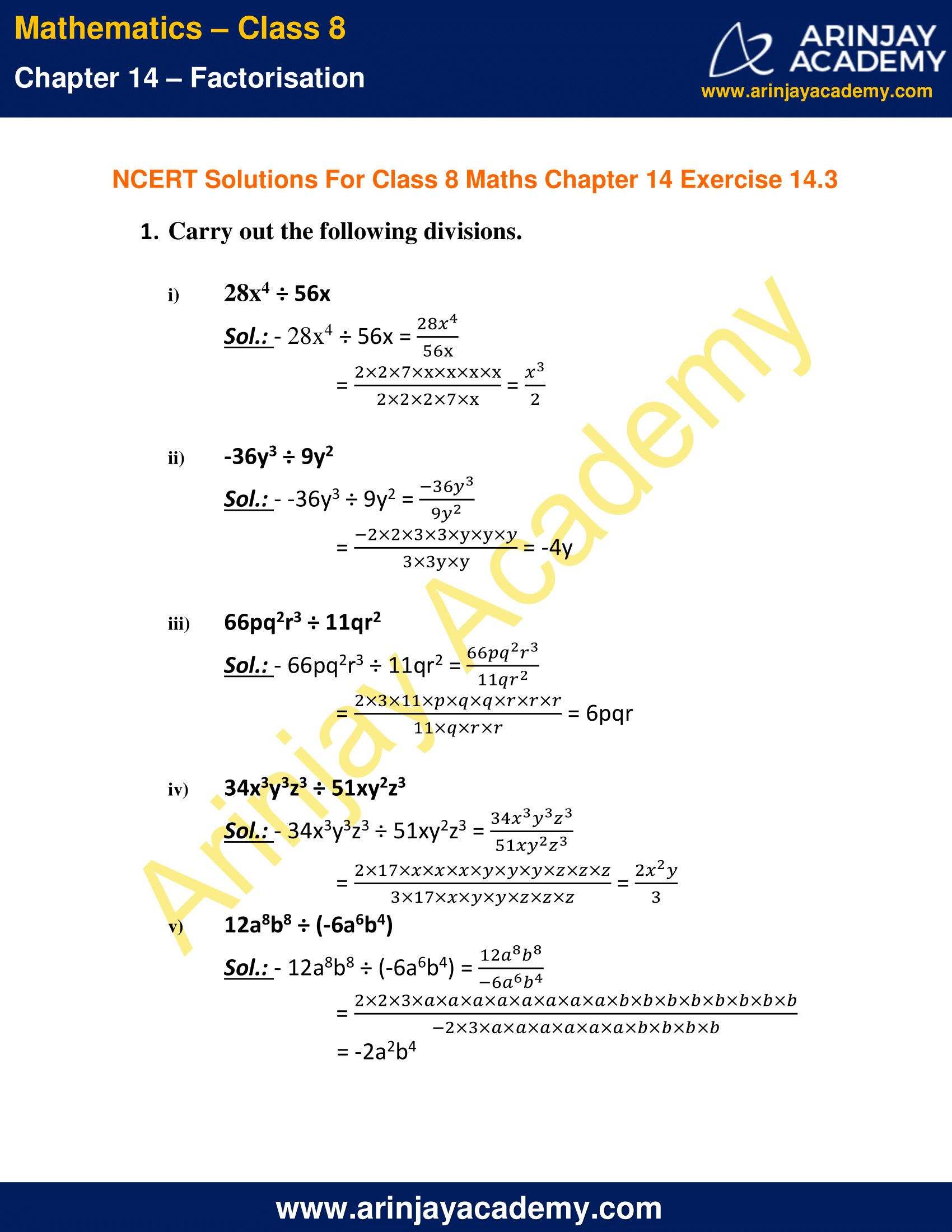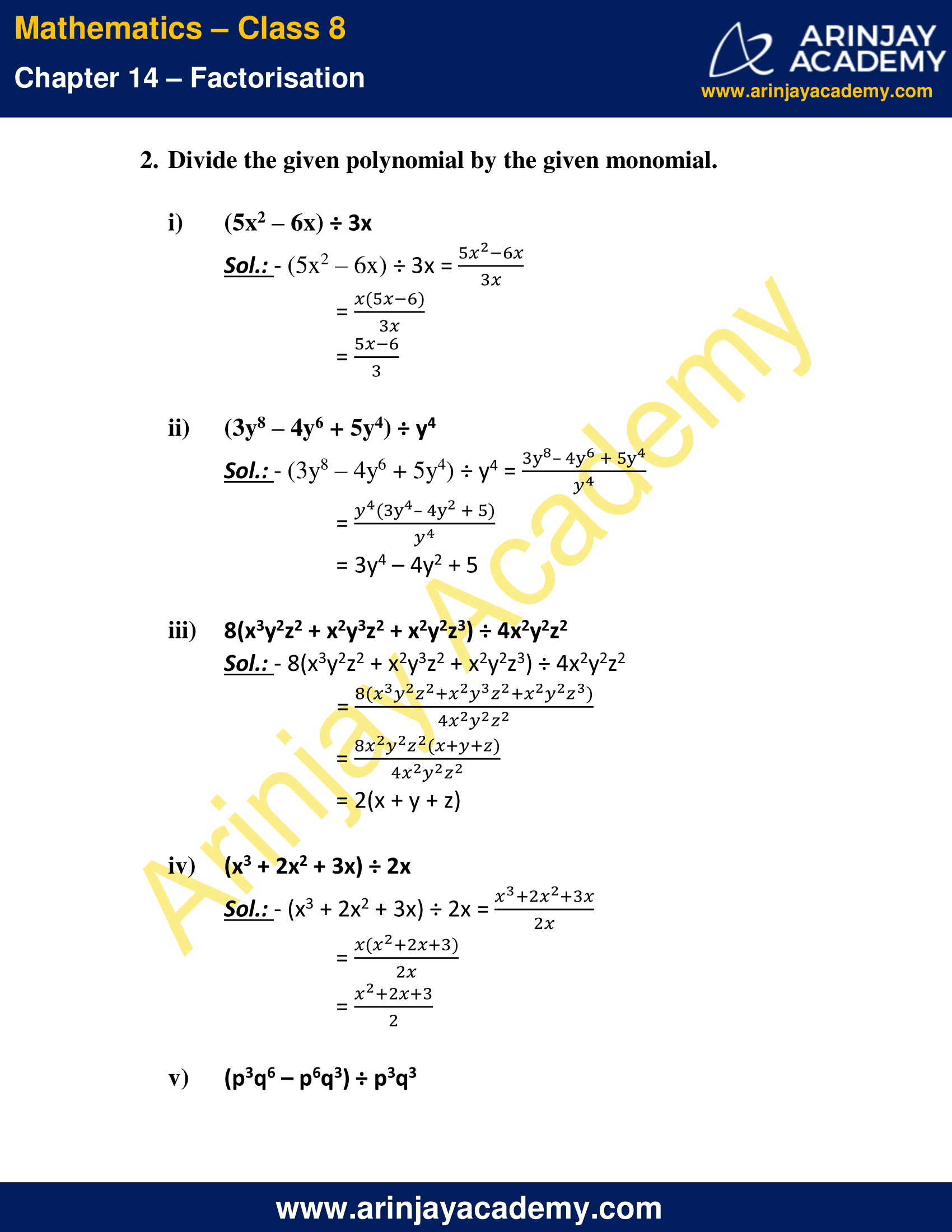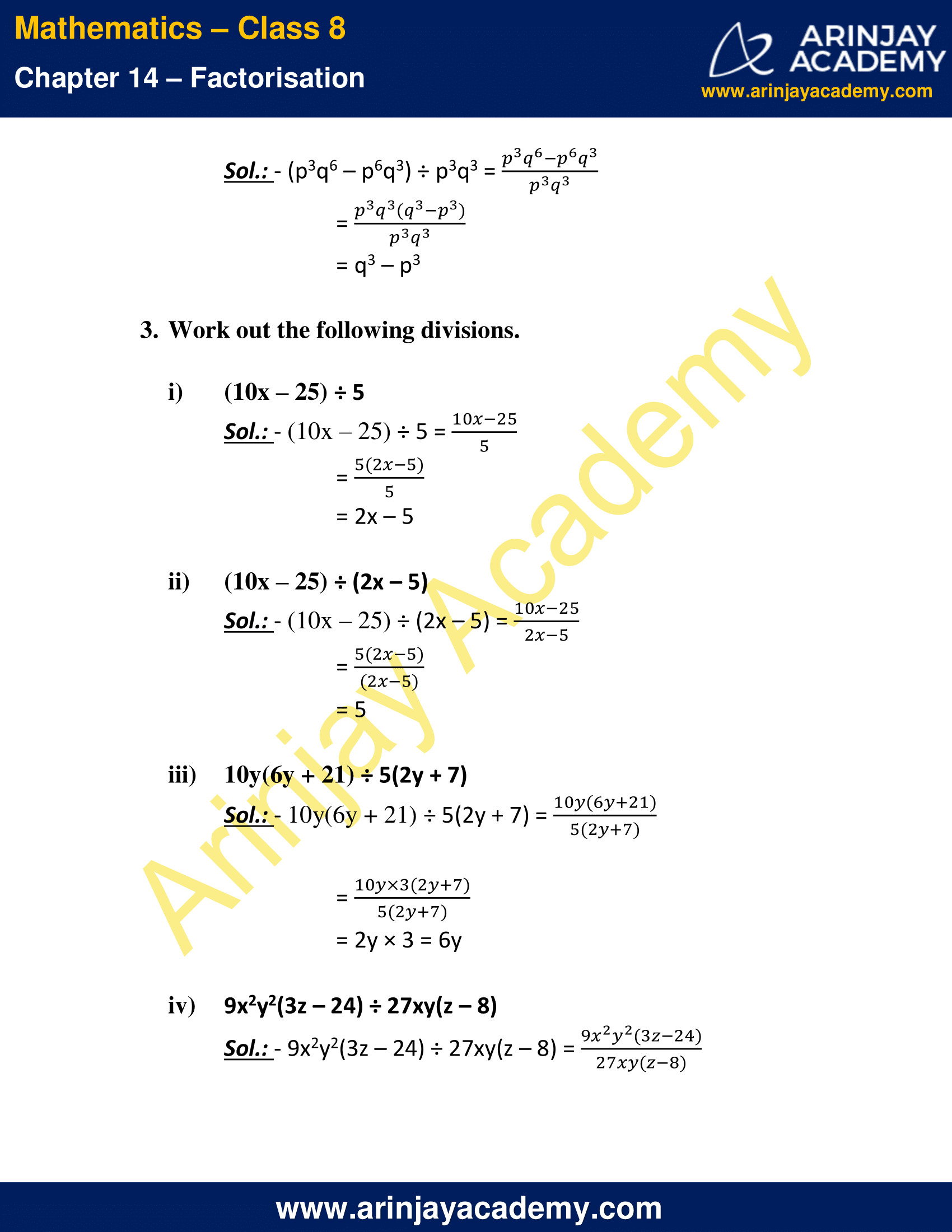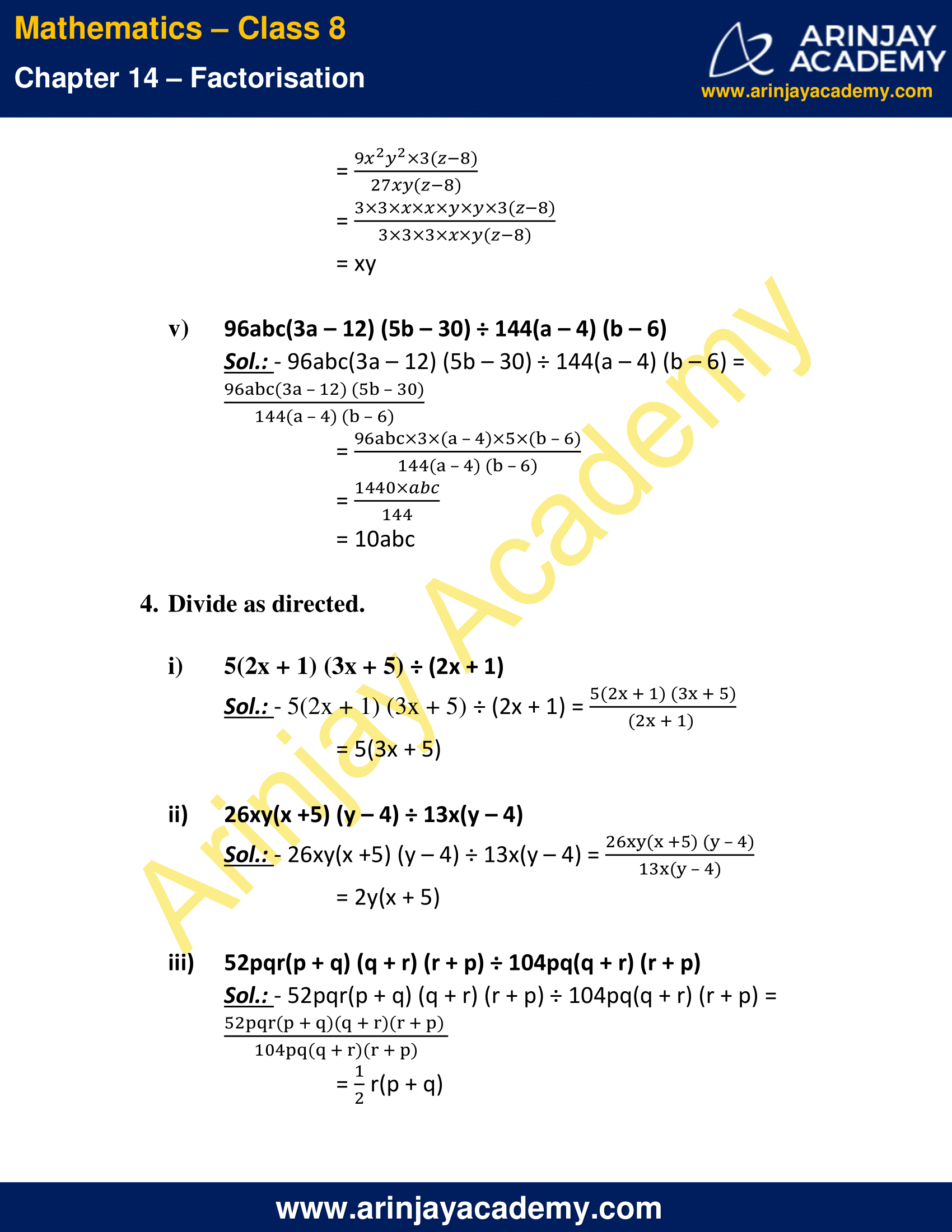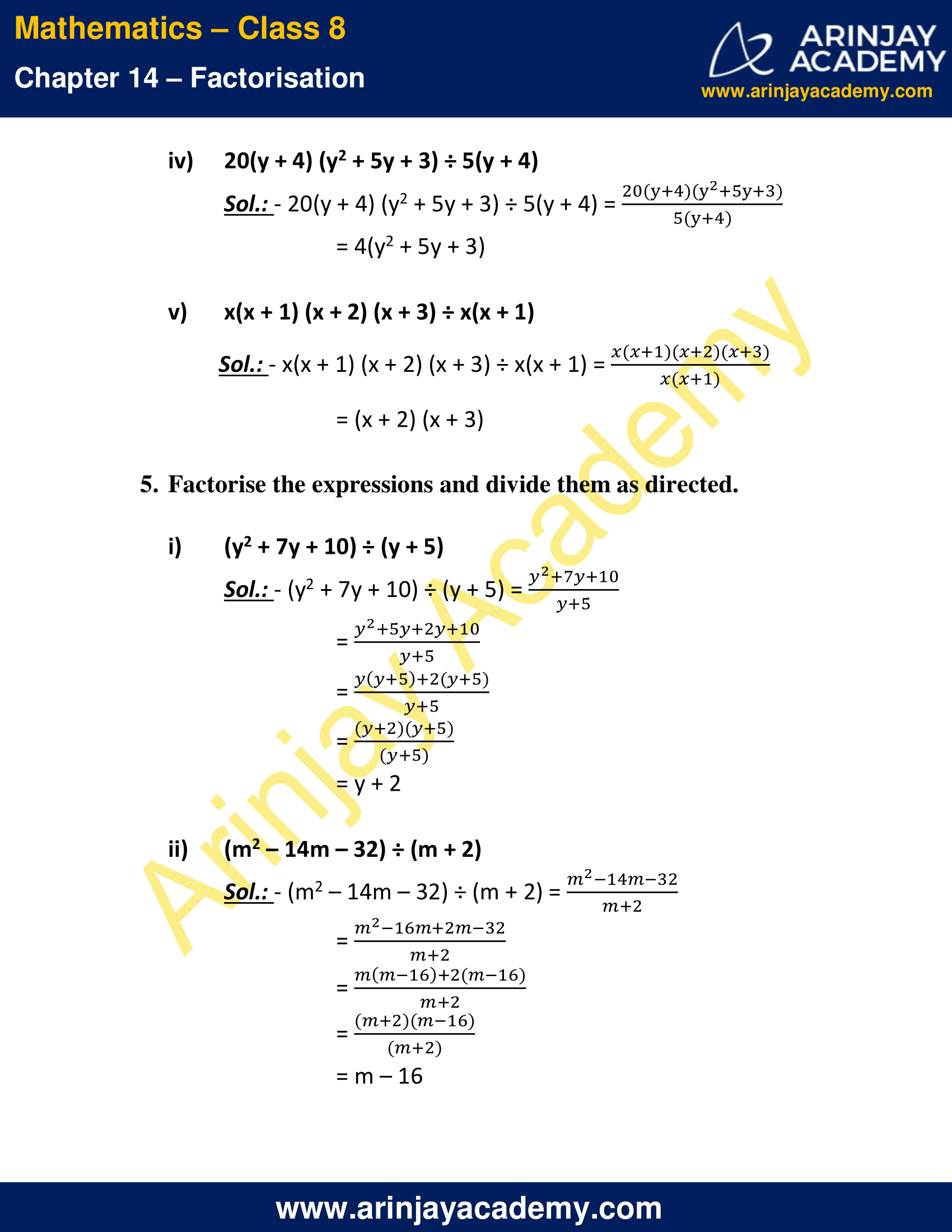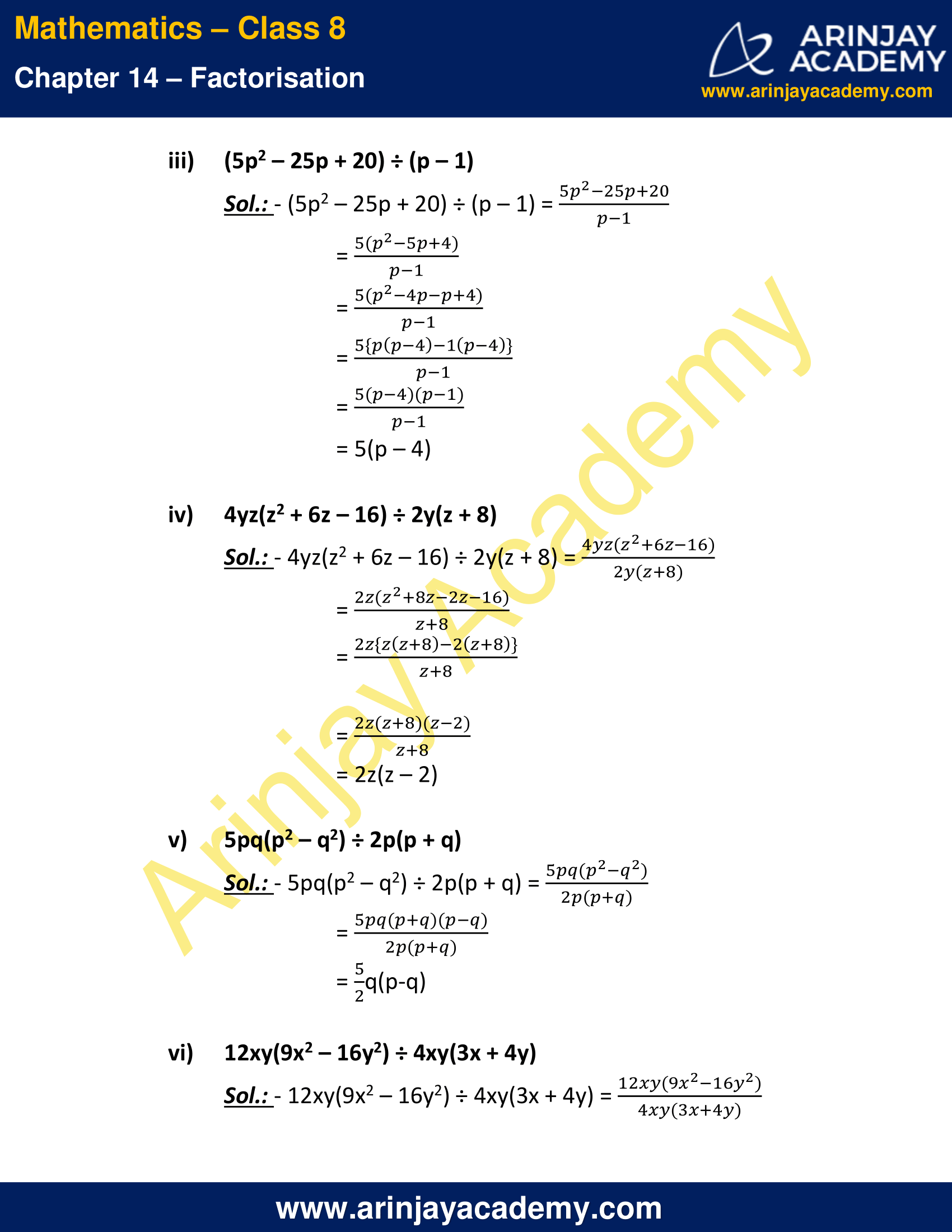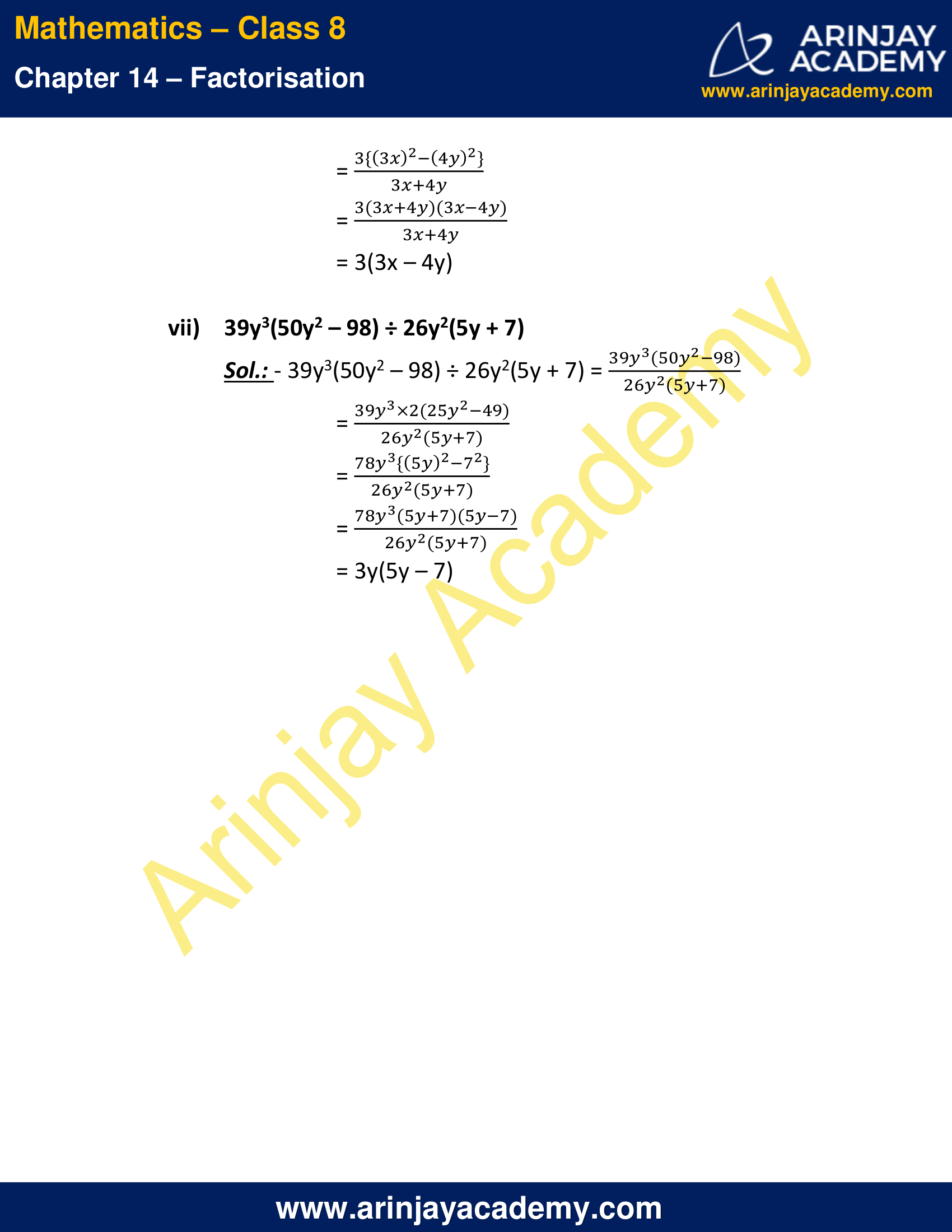The next Exercise for NCERT Solutions for Class 8 Maths Chapter 14 Exercise 14.4 – Factorisation can be accessed by clicking here

Download NCERT Solutions for Class 8 Maths Chapter 14 Exercise 14.3 – Factorisation